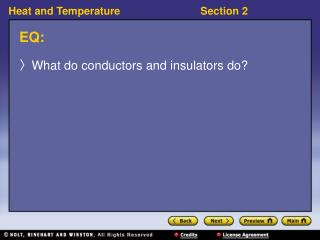DownloadDownload PresentationEQ:

# EQ:

Télécharger la présentation## EQ:

- - - - - - - - - - - - - - - - - - - - - - - - - - - E N D - - - - - - - - - - - - - - - - - - - - - - - - - - -
##### Presentation Transcript

1. EQ: • What do conductors and insulators do?

2. Class Starter. Check EQ and MV. The two pictures all show examples of energy transfer. Answer the questions about what happens in each picture, and identify how the heat goes from one object to another in each case. • Why is it a bad idea to drink hot cocoa out of a tin cup? Explain the energy transfer on the atomic level. 2. What happens to your hand when you place it above a lighted candle? (Assume you are not touching the flame. Explain the energy transfers on the atomic level. Hint: Remember that warm air rises.)

3. Methods of Energy Transfer • How does energy transfer happen? • Heat energy can be transferred in three ways: conduction, convection, and radiation.

4. Methods of Energy Transfer, continued • Conduction occurs between objects in direct contact. • thermal conduction:the transfer of energy as heat through a material • Convection results from the movement of warm fluids. • convection:the movement of matter due to differences in density that are caused by temperature variations • convection current:any movement of matter that result from differences in density; may be vertical, circular, or cyclical • The heating and cooling of a room involve convection currents.

5. Conduction and Convection

6. Methods of Energy Transfer, continued • Radiation does not require physical contact between objects. • radiation:the energy that is transferred as electromagnetic waves, such as visible light and infrared waves • All hot objects give off infrared radiation. • Unlike conduction and convection, radiation does not involve the movement of matter across space. • Radiation is the only way that energy can be transferred through a vacuum • Much of the energy we receive from the sun is transferred by radiation.

7. Conductors and Insulators • What do conductors and insulators do? • A conductor is a material through which energy can be easily transferred as heat. • An insulator is a material that transfers energy poorly.

8. Conductors and Insulators, continued • Heat energy is transferred through particle collisions. • Gases are very poor heat conductors because their particles are so far apart. • Denser materials usually conduct energy better than less dense materials do. • Metals tend to conduct energy very well. • Plastics conduct energy poorly.

9. Specific Heat • What makes something a good conductor of heat? • What makes a substance a good or poor conductor depends in part on how much energy is required to change the temperature of the substance by a certain amount. • specific heat:the quantity of heat required to raise a unit mass of homogenous material 1 K or 1 °C in a specified way given constant pressure and volume

10. Specific Heat, continued • Specific heat describes how much energy is required to raise an object’s temperature. • Specific heat is a characteristic physical property. • It is represented by c. • Specific heat can be used to figure out how much energy it takes to raise an object’s temperature. Specific Heat Equation energy = specific heat  mass  temperature change energy = cmDT

11. Values of Specific Heat at 25 °C

12. Math Skills Specific Heat How much energy must be transferred as heat to 200 kg of water in a bathtub to raise the water’s temperature from 25 °C to 37 °C? 1.List the given and the unknown values. Given: ∆T = 37 °C – 25 °C = 12 °C = 12 K ∆T = 12 K m = 200 kg c = 4,186 J/kg•K Unknown:energy = ? J

13. Math Skills, continued 2. Write down the specific heat equation. energy = cmDT 3. Substitute the specific heat, mass, and temperature change values, and solve.

14. Specific Heat, continued • Heat raises an object’s temperature or changes the object’s state. • While a substance is melting or boiling, the temperature does not change.

15. Visual Concept: Heating Systems

16. Visual Concept: Refrigeration